# Part 1: Part 2: 1 2/2 Calculate lim +0 ( + ) Let f(x) be a...

Part 1: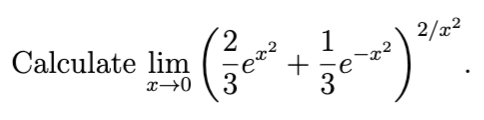Part 2: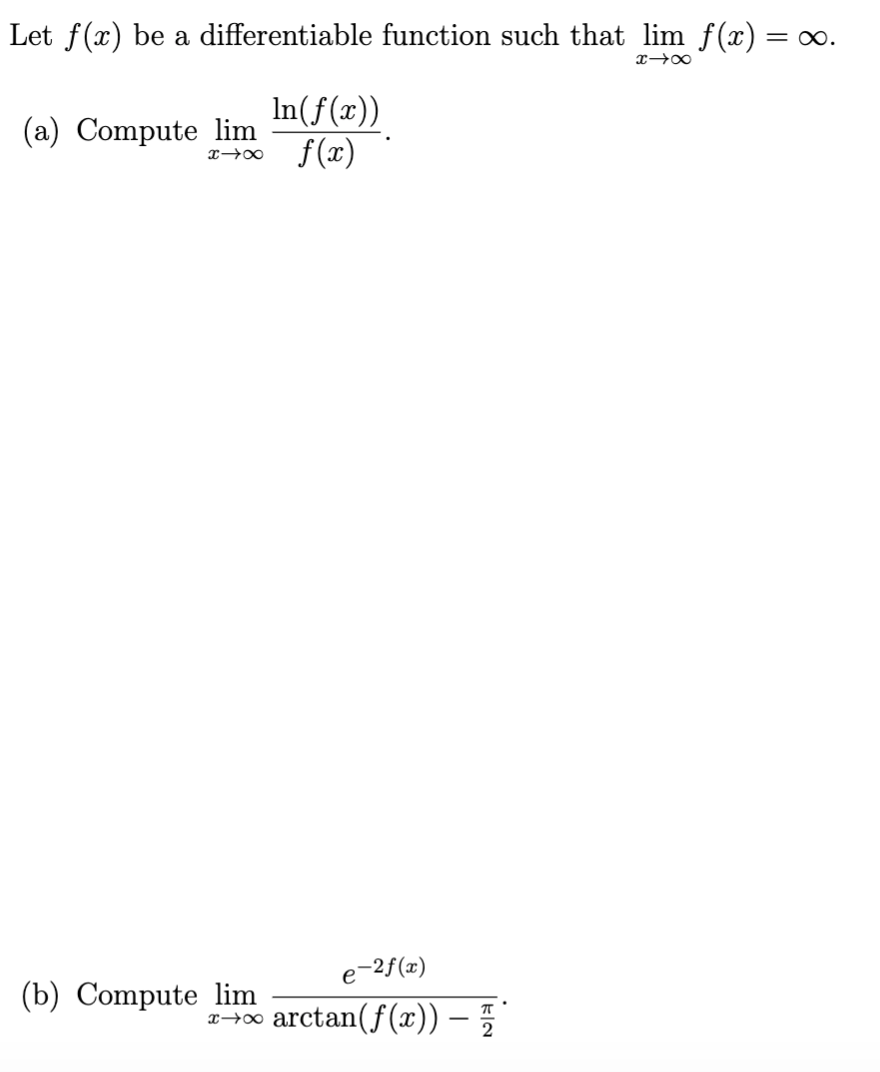1 2/2 Calculate lim +0 ( + )
Let f(x) be a differentiable function such that lim f(x) = 00. 200 In(f(x)) (a) Compute lim 10 f(x) e-2f(x) (b) Compute lim 2400 arctan( f(x)) –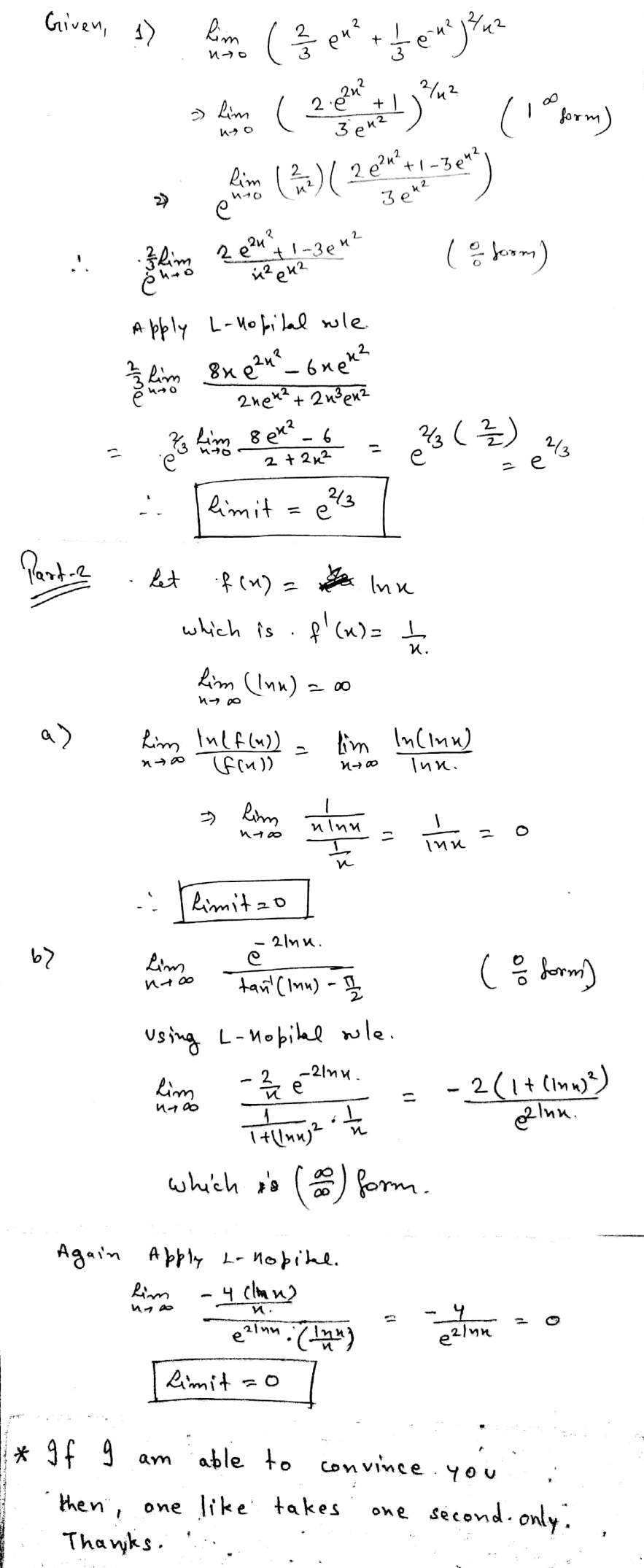#### Earn Coin

Coins can be redeemed for fabulous gifts.

Similar Homework Help Questions
• ### Let f(x) be a differentiable function with inverse of f(x) such that f(0)=0 and f'(0) is not 0. Prove lim(x->0) f...

Let f(x) be a differentiable function with inverse of f(x) such that f(0)=0 and f'(0) is not 0. Prove lim(x->0) f(x)/f −1(x) =f'(0)^2 f-1(x) is f inverse of x

• ### 10. Let f(x)- x+1, when x<0 x21, when x20 Calculate Sro(x) Graph fx) near zero and...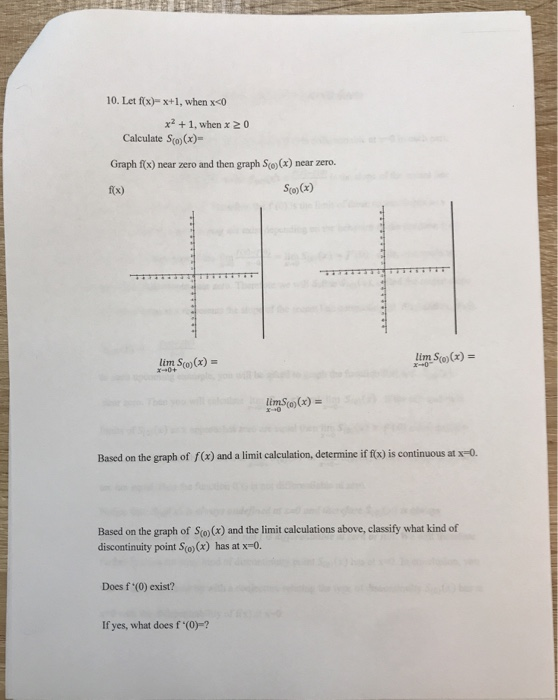10. Let f(x)- x+1, when x<0 x21, when x20 Calculate Sro(x) Graph fx) near zero and then graph Sce)(x) near zero. So)(x) fx) lim So (x) = x 0 lim So)(x) x0+ limSt (x) x0 Based on the graph of f(x) and a limit calculation, deternmine if f(x) is continuous at x=0. Based on the graph of So)(x) and the limit calculations above, classify what kind of discontinuity point S(o) (x) has at x-0 Does f '(0) exist? If yes,...

• ### 2. Let f: R R be a continuous function. Suppose that f is differentiable on R\{0} and that there exists an L e R such that lim,of,(z) = L. Prove that f is differentiable at 1-0 with f,(0) = L. (Hint:...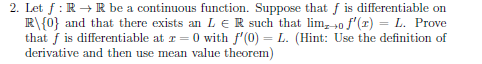2. Let f: R R be a continuous function. Suppose that f is differentiable on R\{0} and that there exists an L e R such that lim,of,(z) = L. Prove that f is differentiable at 1-0 with f,(0) = L. (Hint: Use the definition of derivative and then use mean value theorem) 2. Let f: R R be a continuous function. Suppose that f is differentiable on R\{0} and that there exists an L e R such that lim,of,(z) =...

• ### "Mx Letf: R-> R be a differentiable function and f(15)-2. Then the value of lim x...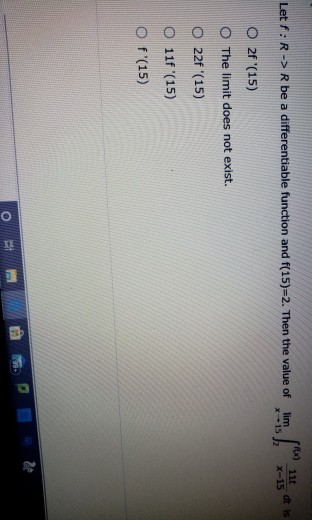"Mx Letf: R-> R be a differentiable function and f(15)-2. Then the value of lim x 15 dt is X-15 O 2f '(15) The limit does not exist. O22f '(15) O 11f '(15) O f'(15) 3 23 o

• ### 12) Let f(A) = x + 1 xxo 0 x=0 (ax+ 2 x>0 a) Find lim...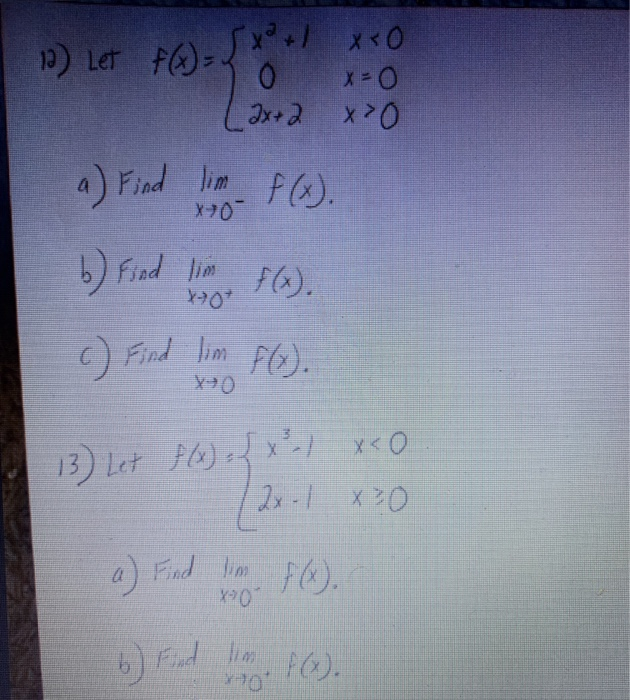12) Let f(A) = x + 1 xxo 0 x=0 (ax+ 2 x>0 a) Find lim f(x). X-70 b) Find lim F(x). X70 c) Find lim f(x). 13) Let f(x) = x - x .O 12.1 x 0 a) Find lim f(x). 6) Find

• ### Q3 (Prove that P∞ k=1 1/kr < ∞ if r > 1) . Let f : (0,∞) → R be a twice differentiable function with f ''(x) ≥ 0 for all x ∈ (0,∞). (a) Show that f '(k) ≤ f(k + 1) − f(k) ≤ f '(...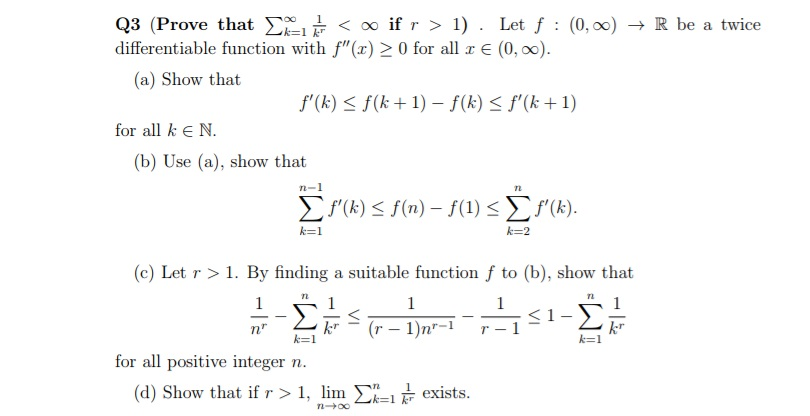Q3 (Prove that P∞ k=1 1/kr < ∞ if r > 1) . Let f : (0,∞) → R be a twice differentiable function with f ''(x) ≥ 0 for all x ∈ (0,∞). (a) Show that f '(k) ≤ f(k + 1) − f(k) ≤ f '(k + 1) for all k ∈ N. (b) Use (a), show that Xn−1 k=1 f '(k) ≤ f(n) − f(1) ≤ Xn k=2 f '(k). (c) Let r > 1. By finding...

• ### I need an explanation using calculus 1 Problem 6 Let f: R R be a differentiable...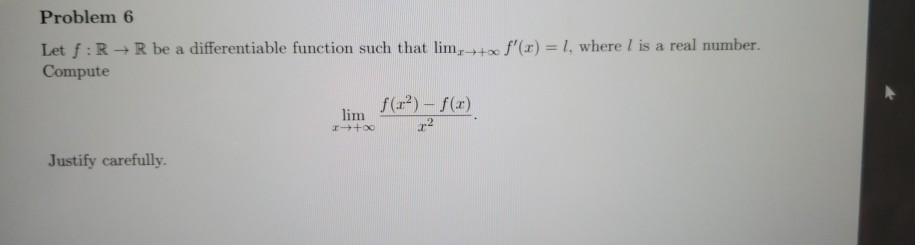I need an explanation using calculus 1 Problem 6 Let f: R R be a differentiable function such that lim, Compute 1, where l is a real number. f'(x) lim )-f(r) Justify carefully. Problem 6 Let f: R R be a differentiable function such that lim, Compute 1, where l is a real number. f'(x) lim )-f(r) Justify carefully.

• ### 2. Let g(x) In f(x) where f(x) is a twice differentiable positive function on (0, o) such that f(x + 1) = x f(x) Then f...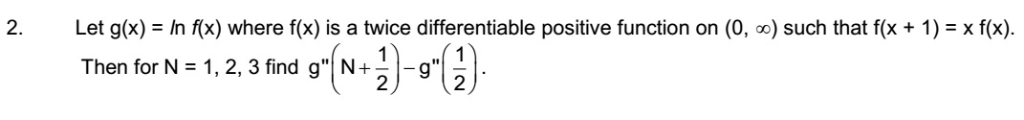2. Let g(x) In f(x) where f(x) is a twice differentiable positive function on (0, o) such that f(x + 1) = x f(x) Then for N 1, 2, 3 find g" N+ 2 2. Let g(x) In f(x) where f(x) is a twice differentiable positive function on (0, o) such that f(x + 1) = x f(x) Then for N 1, 2, 3 find g" N+ 2

• ### Question 1 1. [5 pts] Give a complete definition of lim f(x) = -oo if... 2....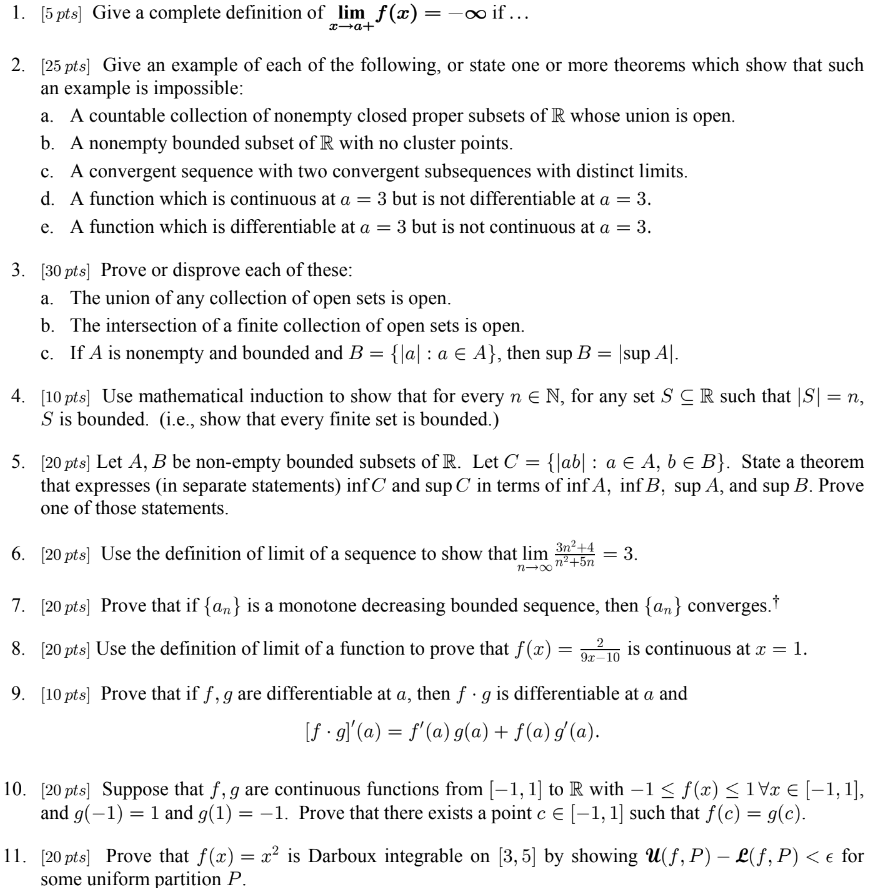Question 1 1. [5 pts] Give a complete definition of lim f(x) = -oo if... 2. [25 pts] Give an example of each of the following, or state one or more theorems which show that such an example is impossible: a. A countable collection of nonempty closed proper subsets of R whose union is open. b. A nonempty bounded subset of R with no cluster points. c. A convergent sequence with two convergent subsequences with distinct limits. d. A function...

• ### Let f be defined on an open interval I containing a point a (1) Prove that if f is differentiable on I and f"(a) exists, then lim h-+0 (a 2 h2 (2) Prove that if f is continuous at a and there...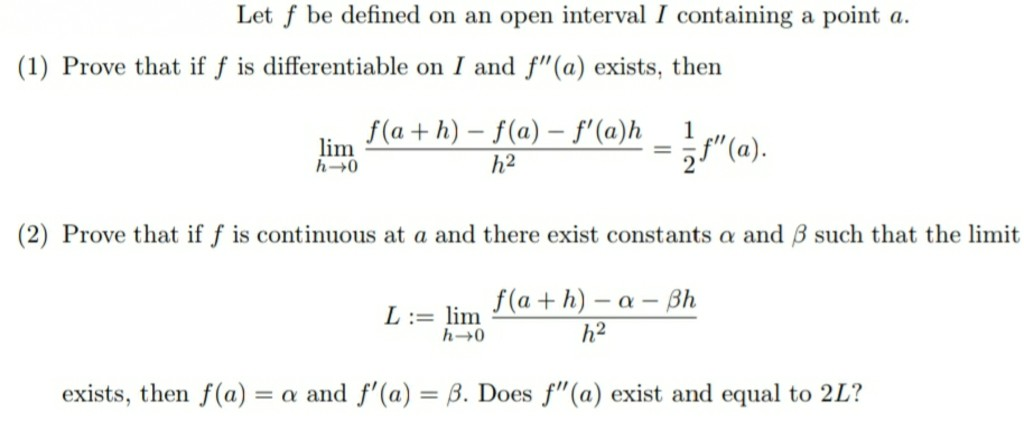Let f be defined on an open interval I containing a point a (1) Prove that if f is differentiable on I and f"(a) exists, then lim h-+0 (a 2 h2 (2) Prove that if f is continuous at a and there exist constants α and β such that the limit L := lim h2 exists, then f(a)-α and f'(a)-β. Does f"(a) exist and equal to 2L? Let f be defined on an open interval I containing a point a...

Free Homework Help App